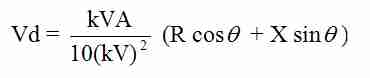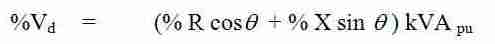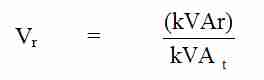Without capacitors, the voltage drop across a system is calculated as::orkV       =          phase to phase voltage at load

cos theta    =    cosine of the power factor angle

X         =          inductive reactance of the system leading up to the load (ohms)

The percent voltage rise at a load due to the addition of a capacitor bank is:

Vr        =          % voltage rise at load

kVAR =          3-phase capacitive kilovar

kV       =          phase to phase kilovolts

X         =          inductive reactance of the system

KVAsc =         3-phase short circuit kVA

The percent voltage rise from primary to secondary of a transformer is:Where:

Vr        =          the percent voltage rise at the transformer

kVAR =          3-phase kilovar applied

kVAt   =          3-phase kVA of the transformer

Xt        =          transformer reactance in percent